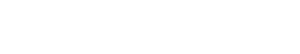# Document topic probabilities

Document topic probabilities are the likelihood that a document falls into a given topic. This is often determined by algorithms, which analyze the text of the document and compare it to a set of known topics. The algorithm then produces a score for each topic, which represents the probability that the document belongs to that topic.

Document topic probabilities can be used for a variety of purposes, including determining which topics are most relevant to a given document, finding documents that are similar to one another, and identifying trends in how topics are used over time.

When disambiguating this term, it is important to note that it is different from topic modelling, which is a technique used to identify the latent topics in a collection of documents. Topic modelling does not produce probabilities, but rather produces a set of topics with associated weights. These weights can be thought of as the probability that a document belongs to a given topic, but they are not the same as document topic probabilities.

Document topic probabilities can be useful for a variety of tasks in text analytics, such as document classification, clustering, and retrieval. They can also be used in combination with other features, such as term frequencies or co-occurrence matrices, to improve the performance of these tasks.

There are a variety of ways to compute document topic probabilities, including statistical methods like latent Dirichlet allocation (LDA) and support vector machines (SVMs), and heuristic methods like word embeddings.

## Document topic probabilities and LDA

Latent Dirichlet allocation (LDA) is a statistical technique that can be used to generate document topic probabilities. It does this by first assigning each document to a mixture of topics, and then estimating the probability of each topic given the text of the document.

LDA is a popular method for generating document topic probabilities, but it has a number of drawbacks. First, it requires a large amount of data in order to produce accurate results. Second, it can be difficult to interpret the results of an LDA analysis. Finally, LDA is computationally intensive, which can make it impractical for large-scale text analytics tasks.

## Document topic probabilities and SVMs

Support vector machines (SVMs) are a type of machine learning algorithm that can be used to compute document topic probabilities. SVMs work by mapping the documents in a dataset onto a high-dimensional space, and then finding a hyperplane that separates the documents into two classes. The hyperplane is then used to calculate the probability that a new document belongs to each class.

SVMs have a number of advantages over other methods for computing document topic probabilities. First, they can be trained on small datasets and still produce accurate results. Second, they are relatively fast, which makes them well suited for large-scale text analytics tasks. Third, SVMs can be used with a variety of different feature representations, including term frequencies and co-occurrence matrices.

## Document topic probabilities and heuristic methods

Heuristic methods are a type of algorithm that makes decisions based on a set of rules, rather than on data. Heuristic methods are often used when data is not available, or when it is not possible to use a more traditional method like an SVM.

One common heuristic method for computing document topic probabilities is word embeddings. Word embeddings are a type of vector representation that encodes the meaning of words in a high-dimensional space. This space can then be used to calculate the similarity between documents, which can be used to infer the probability that a document belongs to a given topic.

Word embeddings have a number of advantages over other methods for computing document topic probabilities. First, they do not require a large amount of data in order to produce accurate results. Second, they are relatively fast, which makes them well suited for large-scale text analytics tasks. Finally, word embeddings can be used with a variety of different feature representations, including term frequencies and co-occurrence matrices.Unlock the power of actionable insights with AI-based natural language processing.